# Rhombus

Calculate the perimeter and area of ​​a rhombus whose diagonals are 39 cm and 51 cm long.

Result

p =  128.406 cm
S =  994.5 cm2

#### Solution:

$u_{ 1 } = 39 \ cm \ \\ u_{ 2 } = 51 \ cm \ \\ \ \\ a^2 = (u_{ 1 }/2)^2+(u_{ 2 }/2)^2 \ \\ a = \sqrt{ (u_{ 1 }/2)^2+(u_{ 2 }/2)^2 } = \sqrt{ (39/2)^2+(51/2)^2 } \doteq 32.1014 \ cm \ \\ \ \\ p = 4 \cdot \ a = 4 \cdot \ 32.1014 = 6 \ \sqrt{ 458 } \doteq 128.4056 = 128.406 \ \text{ cm }$
$S = \dfrac{ u_{ 1 } \cdot \ u_{ 2 } }{ 2 } = \dfrac{ 39 \cdot \ 51 }{ 2 } = \dfrac{ 1989 }{ 2 } = 994.5 = 994.5 \ cm^2$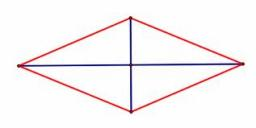Our examples were largely sent or created by pupils and students themselves. Therefore, we would be pleased if you could send us any errors you found, spelling mistakes, or rephasing the example. Thank you!

Leave us a comment of this math problem and its solution (i.e. if it is still somewhat unclear...):Be the first to comment!Tips to related online calculators
Pythagorean theorem is the base for the right triangle calculator.

## Next similar math problems:

1. Diamond perimeter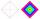Calculate the diamond circumference which area is 288 cm square and one diagonal has a size of 124 cm.
2. Diamond and diagonalsA diamond has diagonals f = 8 cm and g = 6 cm long. How long is this diamond perimeter? (Calculate it!)
3. RhombusCalculate the length of the diagonal AC of the rhombus ABCD, if its perimeter is 84 dm and the other diagonal BD has length 20 dm.
4. Rhombus IVCalculate the length of the diagonals of the rhombus, whose lengths are in the ratio 1: 2 and a rhombus side is 35 cm.
5. Diagonals in diamons/rhombusRhombus ABCD has side length AB = 4 cm and a length of one diagonal of 6.4 cm. Calculate the length of the other diagonal.
6. Diamond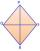Calculate the length of the two diagonals of the diamond if: a = 13 cm v = 12 cm
7. Park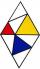In the park is marked diamond shaped line connecting locations A, D, S, C, B, A. Calculate its length if |AB| = 108 m, |AC| = 172.8 m.
8. Diagonals of diamondFind the area and circumference of the diamond ABCD with 15m and 11m diagonals.
9. DiamondDetermine the side of diamond if its content is S = 353 cm2 and one diagonal u2 = 45 cm.
10. DiagonalsA diagonal of a rhombus is 20 cm long. If it's one side is 26 cm find the length of the other diagonal.
11. EstateEstate shaped rectangular trapezoid has bases long 34 m , 63 m and perpendicular arm 37 m. Calculate how long is its fence.
12. Concentric circlesIn the circle with diameter 19 cm is constructed chord 9 cm long. Calculate the radius of a concentric circle that touches this chord.
13. Tree trunkFrom the tree trunk, the diameter at the narrower end is 28 cm, a beam of square cross-section is to be made. Calculate the longest side of the largest possible square cross-section.
14. ISO trapezoid v2bases of Isosceles trapezoid measured 12 cm and 5 cm and its perimeter is 21 cm. What is the are of a trapezoid?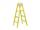The double ladder has 3 meters long shoulders. What is the height of the upper of the ladder reach if the lower ends are 1.8 meters apart?Sanusha buys a piece of satin 2.4 m wide. The diagonal length of the fabric is 4m. What is the length of the piece of satin?The size of a tv screen is given by the length of its diagonal. If the dimension of a tv screen is 16 inches by 14 inches, what is the size of the tv screen?